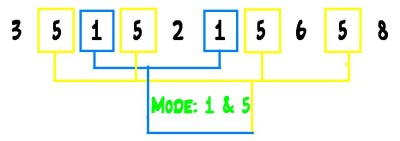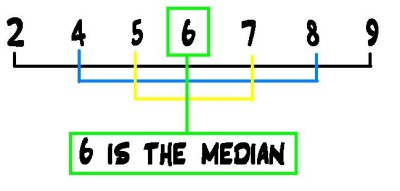List of public pages created with Protopage

Home

Plain sticky notes

Sticky note

What is algerbra ? The part of mathematics in which letters and other symbols are used to represent numbers and quantities in formulae and equations.

Todo lists

Todo listRevise atleast 2-3hours a weekBuy the maths revision booksPractice the subjects in algerbra that you find the most difficultMaybe watch videos on how to d certain parts of algerbra ( some people find that easier than writing it down

Photos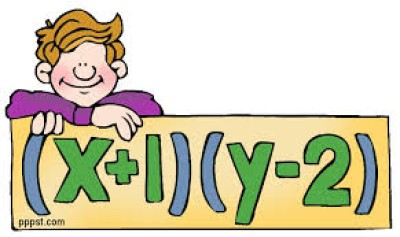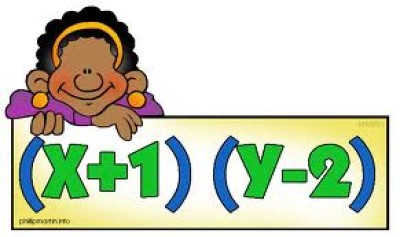REVISION

Plain sticky notes

How to revise

Different ways of revising - If you are able to access the interent you will be able to research online a certain topic that you stuggled the most with. -(Again you need internet for this.) you can watch "how to" videos on again the topics in which you wish to improve on, the most. -There are also many ways to revise without the guidence of the internet you can also by revision books. -You can do your own revision book and always keep it with you , so if you get stuck of a certain question you can just look in your revidion book. -Finally you can just uses your math book to help you and get your maths teacher to write percific notes in it that you think will help you the most.

Sticky note

Steps you have to take before revising... 1. Before you actually start to revise you need to have a plan in your head on what subject you are going to focus on and also for how long you Will focus on each subject. 2. You can maybe set a target or a treat for you so that you are motivated to do more work.And then you will get the treat or reward after you hsve finished your revision for the week or day. 3.Never just focus on things from textbooks.Or the internet , try to create a rvision book so you can focos on that. Or you can even ask your teacher to create a revision book for you when you are in class. 4. NEVER limit yourself to just one topic that you struggle with. 5. Try not to use a calculator unless you are really struggerling with the question or equation. 6. My final piece of advice is to NEVER get too stressed before you are doing a test or exam because this may cause you to panic and forget what ou had been revising previously.

PhotosSubstitution

Plain sticky notes

Sticky note

RULE Adding or Subtracting Multiplying or Dividing Two like signs become a positive sign 3+(+2) = 3 + 2 = 5 3 × 2 = 6 6-(-3) = 6 + 3 = 9 (-3) × (-2) = 6 Two unlike signs become a negative sign 7+(-2) = 7 - 2 = 5 3 × (-2) = -6 8-(+2) = 8 - 2 = 6 (-3) × 2 = -6

Sticky note

In Algebra "Substitution" means putting numbers where the letters are: If you have -------> x - 2 and you know that ---------> x = 6 then you can "substitute" 6 for x ----------> 6 - 2 = 4

Photos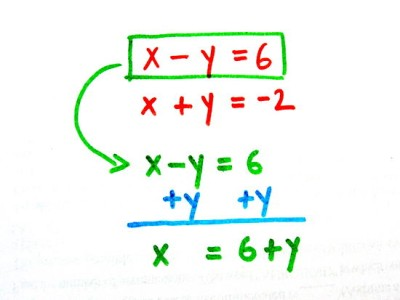Solving Linear equations

Plain sticky notes

Sticky note

This Powerpoint is an extremely using tool to use if you have trouble learning how to use and solve A Linear Equations. It was able to help me a awful lot , it help me to understand which part of the equation that you have to divide and multiply. I also struggled to get the grasp of the final thing that you had to do , but now i am fine with the whole consept.

Factorising

Plain sticky notes

Sticky note

Factorising Quadratics There is no simple method of factorising a quadratic expression, but with a little practise it becomes easier. One systematic method, however, is as follows: Example Factorise 12y² - 20y + 3 = 12y² - 18y - 2y + 3 [here the 20y has been split up into two numbers whose multiple is 36. 36 was chosen because this is the product of 12 and 3, the other two numbers]. The first two terms, 12y² and -18y both divide by 6y, so 'take out' this factor of 6y. 6y(2y - 3) - 2y + 3 [we can do this because 6y(2y - 3) is the same as 12y² - 18y] Now, make the last two expressions look like the expression in the bracket: 6y(2y - 3) -1(2y - 3) The answer is (2y - 3)(6y - 1) Example Factorise x² + 2x - 8 We need to split the 2x into two numbers which multiply to give -8. This has to be 4 and -2. x² + 4x - 2x - 8 x(x + 4) - 2x - 8 x(x + 4)- 2(x + 4) (x + 4)(x - 2) - See more at: http://www.mathsrevision.net/factorising#sthash.JSRDls2j.dpuf

Sticky note

Expanding Brackets Brackets should be expanded in the following ways: For an expression of the form a(b + c), the expanded version is ab + ac, i.e., multiply the term outside the bracket by everything inside the bracket (e.g. 2x(x + 3) = 2x² + 6x [remember x × x is x²]). For an expression of the form (a + b)(c + d), the expanded version is ac + ad + bc + bd, in other words everything in the first bracket should be multiplied by everything in the second. Example Expand (2x + 3)(x - 1): (2x + 3)(x - 1) = 2x² - 2x + 3x - 3 = 2x² + x - 3 - See more at: http://www.mathsrevision.net/factorising#sthash.JSRDls2j.dpuf

Todo lists

Todo listIf you still are finding these subjects difficult then just go on mymaths or any other revision website like bitesize for example

Bookmarks

Plain sticky notes

Sticky note

Click 'edit' on the Bookmarks widget to add all of your favorite sites to your page for quick access

simplifying algebraic expressions

Plain sticky notes

Sticky note

This is a great tool to use if you are having soom trouble with a equation that you need to simplify ---------------------------------------------------------------------->

Plain sticky notes

Sticky note

A Rhyme To Help You Remember ♫ "If adding or subtracting is your aim, The bottom numbers must be the same! ♫ "Change the bottom using multiply or divide, But the same to the top must be applied, ♫ "And don't forget to simplify, Before its time to say good bye"

Sticky note

For your exam, you will need to feel confident when dealing with fractions. This section will help you to recognise equivalent fractions and practise simple calculations. Adding and subtracting fractions added fraction It is easy to add fractions when the numbers on the bottom are the same. All you need to do is add the tops of the fractions together. So 2/9 + 5/9 = 7/9 Sometimes you need to cancel down the answer to its simplest terms. 3/10 + 1/10 = 4/10 = 2/5 When the numbers on the bottom are not the same to start with, you use equivalent fractions to make them the same.

Photos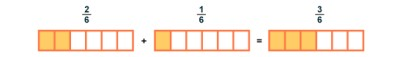Multiplying Fractions

Plain sticky notes

Sticky note

There are 3 simple steps to multiply fractions 1. Multiply the top numbers (the numerators). 2. Multiply the bottom numbers (the denominators). 3. Simplify the fraction if needed.

Mode,Median and mean

Plain sticky notes

Mode

You can basically say one word to know what you have to work out the mode, and that word is the MOST ---------------------------------------------------------------------------------------------------->

Median

Median is just as easy to work out as the MODE, basically what you have to do is just lay your number sequence out in the order of smallest to biggest, once you have done that you then run through the numbers until you get to the middle onces. If you get in the pradicament were you have two numbers in the middle what you have to do if add them together and divide them by two ( becasue there is two of them.There will NEVER be more that 1 or 2 in the middle. -------------------------------------------------------------------------------------------------->

Photos